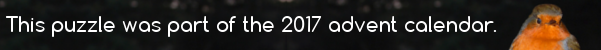mscroggs.co.uk
mscroggs.co.uksubscribe

# Advent calendar 2017

## Archive

Show me a random puzzle
▼ show ▼

## 6 December$$p(x)$$ is a quadratic with real coefficients. For all real numbers $$x$$,
$$x^2+4x+14\leq p(x)\leq 2x^2+8x+18$$
$$p(2)=34$$. What is $$p(6)$$?
© Matthew Scroggs 2019